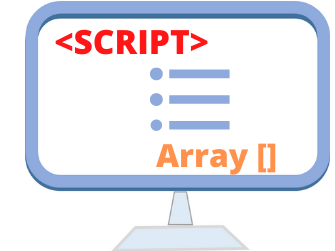# Displaying all elements of an arrayWe used one for loop to display all elements of an array by using their index. To identify how many loops we have to make we must know the total number of elements present inside the array. That we can find out like this

``array_name.length``
So this number we will set as upper limit of our number of rotation or looping.
• ### Displaying elements of an Array

You can check our tutorial on creating array to create an array and now we will try to display each element of the array. Here is the code.
``````var scripts = new Array();
scripts = "PHP";
scripts = "ASP";
scripts = "JavaScript";
scripts = "HTML";

for (i=0;i<scripts.length;i++)
{
document.write(scripts[i] + "<br >");
}``````
The for loop in the above code loops through the elements of the array and display one by one vertically by adding one line break at the end of each element. The upper limit of the for loop is set to script.length value which is in this case equal to 4
scripts is the first element of the array and scripts is the last or 4th element of the array.

## Using toString()

``````<script>
var scripts = new Array();
scripts = "PHP";
scripts = "ASP";
scripts = "JavaScript";
scripts = "HTML";

document.write( scripts.toString());
</script>``````
Output is
``PHP,ASP,JavaScript,HTML``

## Using Join

``````
<script type="text/javascript">
var scripts = new Array();
scripts = "PHP";
scripts = "ASP";
scripts = "JavaScript";
scripts = "HTML";
var str=scripts.join(" : ");
document.write(str);
//document.write(scripts.join(" <br> "));
</script>
``````
The output of the above code is here
``PHP : ASP : JavaScript : HTML ``

## Displaying single element of an array

By using key we can display any element of an array.
``document.write(scripts); // Output is JavaScript``

## Returning Last element of the Array

The first element starts from 0 hence to show the last element of the array we have subtract one from total number of elements present in the array.
``````document.write(scripts[scripts.length-1]); // Output is HTML
``````

## Returning First element of the Array

``````document.write(scripts); // Output is PHP
``````

## Returning all elements with Key

``````<script>
var scripts = new Array();
scripts = "PHP";
scripts = "ASP";
scripts = "JavaScript";
scripts = "HTML";

for (var key in scripts) {
document.write("key : " + key + "  =>value: " + scripts[key] + "<br>");
}
</script>``````
Output
``````key : 0 =>value: PHP
key : 1 =>value: ASP
key : 2 =>value: JavaScript
key : 3 =>value: HTML``````## Subscribe

* indicates required
Subscribe to plus2netplus2net.com